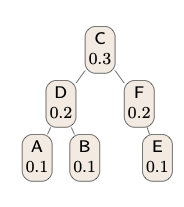## neal young / Chrobak22Huang

• Acta Informatica:21 pages(2022)Huang and Wong  proposed a polynomial-time dynamic-programming algorithm for computing optimal generalized binary split trees. We show that their algorithm is incorrect. Thus, it remains open whether such trees can be computed in polynomial time. Spuler [11, 12] proposed modifying Huang and Wong's algorithm to obtain an algorithm for a different problem: computing optimal two-way-comparison search trees. We show that the dynamic program underlying Spuler's algorithm is not valid, in that it does not satisfy the necessary optimal-substructure property and its proposed recurrence relation is incorrect. It remains unknown whether the algorithm is guaranteed to compute a correct overall solution.